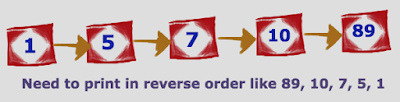Showing posts with label How to print singly linked list in reverse order. Show all posts
Showing posts with label How to print singly linked list in reverse order. Show all posts## How to print singly linked list in reverse order

If we talk about Singly Linked List then it will be a 1 way traversal from head node to tail node. But if we need to print the linked list values from tail node to head node (in reverse order) with O(N) time complexity. ???

• Its simple just we need to apply recursive algorithm where we need to start from head node and reach tail which is last node.
• Next print each node values in recursive call. By this way we can achieve printing linked list in recursive order without traversing more than once which is by time complexity O(N) only.
Lets see simple code to create Singly Linked List and printing same Linked List in reverse order with O(N) complexity.

```class NODE {

int data;
NODE next;

public NODE(int data) {
this.data = data;
this.next = null;
}
}

public class PrintLinkedList {

public static void main(String[] args) {

int val[] = new int[] { 1, 5, 7, 10, 89 };

}

/*
* Recursive method to print LL in reverse order
*/
public void printLLInReverse(NODE tmp) {
if (tmp != null) {
printLLInReverse(tmp.next);
System.out.println(tmp.data);
}
}

/*
* Create linked list based on given array
*/
public NODE createLinkedList(int val[]) {
NODE start = null;
for (int i : val) {
NODE tmp = new NODE(i);
if (start == null) {
start = tmp;
} else {
NODE mover = start;
while (mover.next != null) {
mover = mover.next;
}
mover.next = tmp;
}
}
return start;
}
}
```

OUTPUT:

```89
10
7
5
1
```